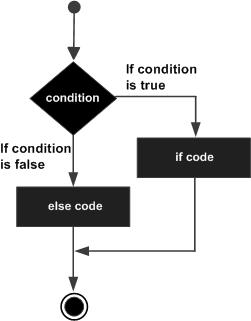## Pascal - if-then-else Statement

Contents[Hide]

An if-then statement can be followed by an optional else statement, which executes when the Boolean expression is false.

## 1. Syntax:

Syntax for the if-then-else statement is:

```if condition then S1 else S2;
```

Where, S1 and S2 are different statements. Please note that the statement S1 is not followed by a semicolon. In the if-then-else statements, when the test condition is true, the statement S1 is executed and S2 is skipped; when the test condition is false, then S1 is bypassed and statement S2 is executed.

For example,

```if color = red then
writeln('You have chosen a red car')else
```

If the boolean expression condition evaluates to true then the if-then block of code will be executed otherwise the else block of code will be executed.

Pascal assumes any non-zero and non-nill values as true and if it is either zero or nill then it is assumed as false value.

## 2. Flow Diagram:## 3. Example 1:

Let us try a complete example that would illustrate the concept:

```program ifelseChecking;
var
{local variable definition }
a : integer;
begin
a :=100;
(* check the boolean condition *)
if( a 20)then
(*if condition istruethenprint the following *)
writeln('a is less than 20')
else
(*if condition isfalsethenprint th following *)
writeln('a is not less than 20');
writeln('value of a is : ', a);
end.
```

When the above code is compiled and executed, it produces following result:

```a is not less than 20
value of a is : 100
```

## 4. The if-then-else if-then-else Statement

An if-then statement can be followed by an optional else if-then-else statement, which is very useful to test various conditions using single if-then-else if statement.

When using if-then , else if-then , else statements there are few points to keep in mind.

• An if-then statement can have zero or one else's and it must come after any else if's.
• An if-then statement can have zero to many else if's and they must come before the else.
• Once an else if succeeds, none of the remaining else if's or else's will be tested.
• No semicolon (;) is given before the last else keyword, but all statements can be compound statements.

## 5. Syntax:

The syntax of an if-then-else if-then-else statement in Pascal programming language is:

```if(boolean_expression 1)then
S1 (*Executeswhen the boolean expression 1istrue*)elseif( boolean_expression 2)then
S2 (*Executeswhen the boolean expression 2istrue*)elseif( boolean_expression 3)then
S3 (*Executeswhen the boolean expression 3istrue*)else
S4;(* executes when the none of the above condition istrue*)
```

## 6. Example 2:

The following example illustrates the concept:

```program ifelse_ifelseChecking;
var
{local variable definition }
a : integer;
begin
a :=100;
(* check the boolean condition *)
if(a =10)  then
(*if condition istruethenprint the following *)
writeln('Value of a is 10')
elseif( a =20)then
(*ifelseif condition istrue*)
writeln('Value of a is 20')
elseif( a =30)then
(*ifelseif condition istrue  *)
writeln('Value of a is 30')
else
(*if none of the conditions istrue*)
writeln('None of the values is matching');
writeln('Exact value of a is: ', a );
end.
```

When the above code is compiled and executed, it produces following result:

```None of the values is matching
Exact value of a is: 100

```